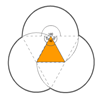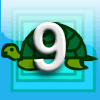# Search by Topic

#### Resources tagged with Angle properties of polygons similar to All about Ratios:

Filter by: Content type:
Age range:
Challenge level:

### There are 22 results

Broad Topics > Angles, Polygons, and Geometrical Proof > Angle properties of polygons### A Sameness Surely

##### Age 14 to 16 Challenge Level:

Triangle ABC has a right angle at C. ACRS and CBPQ are squares. ST and PU are perpendicular to AB produced. Show that ST + PU = AB### Making Sixty

##### Age 14 to 16 Challenge Level:

Why does this fold create an angle of sixty degrees?### Pentakite

##### Age 14 to 18 Challenge Level:

ABCDE is a regular pentagon of side length one unit. BC produced meets ED produced at F. Show that triangle CDF is congruent to triangle EDB. Find the length of BE.### Golden Triangle

##### Age 16 to 18 Challenge Level:

Three triangles ABC, CBD and ABD (where D is a point on AC) are all isosceles. Find all the angles. Prove that the ratio of AB to BC is equal to the golden ratio.### Pent

##### Age 14 to 18 Challenge Level:

The diagram shows a regular pentagon with sides of unit length. Find all the angles in the diagram. Prove that the quadrilateral shown in red is a rhombus.### Tricircle

##### Age 14 to 16 Challenge Level:

The centre of the larger circle is at the midpoint of one side of an equilateral triangle and the circle touches the other two sides of the triangle. A smaller circle touches the larger circle and. . . .### Darts and Kites

##### Age 14 to 16 Challenge Level:

Explore the geometry of these dart and kite shapes!### Dodecawhat

##### Age 14 to 16 Challenge Level:

Follow instructions to fold sheets of A4 paper into pentagons and assemble them to form a dodecahedron. Calculate the error in the angle of the not perfectly regular pentagons you make.### Triangles and Petals

##### Age 14 to 16 Challenge Level:

An equilateral triangle rotates around regular polygons and produces an outline like a flower. What are the perimeters of the different flowers?### Arclets Explained

##### Age 11 to 16

This article gives an wonderful insight into students working on the Arclets problem that first appeared in the Sept 2002 edition of the NRICH website.### At a Glance

##### Age 14 to 16 Challenge Level:

The area of a regular pentagon looks about twice as a big as the pentangle star drawn within it. Is it?##### Age 14 to 16 Challenge Level:

Make five different quadrilaterals on a nine-point pegboard, without using the centre peg. Work out the angles in each quadrilateral you make. Now, what other relationships you can see?### LOGO Challenge 4 - Squares to Procedures

##### Age 11 to 16 Challenge Level:

This LOGO Challenge emphasises the idea of breaking down a problem into smaller manageable parts. Working on squares and angles.### Logo Challenge 3 - Star Square

##### Age 7 to 16 Challenge Level:

Creating designs with squares - using the REPEAT command in LOGO. This requires some careful thought on angles### Angles in Three Squares

##### Age 11 to 16 Challenge Level:

Drawing the right diagram can help you to prove a result about the angles in a line of squares.##### Age 11 to 16 Challenge Level:

Draw some quadrilaterals on a 9-point circle and work out the angles. Is there a theorem?### Semi-regular Tessellations

##### Age 11 to 16 Challenge Level:

Semi-regular tessellations combine two or more different regular polygons to fill the plane. Can you find all the semi-regular tessellations?### No Right Angle Here

##### Age 14 to 16 Challenge Level:

Prove that the internal angle bisectors of a triangle will never be perpendicular to each other.### First Forward Into Logo 7: Angles of Polygons

##### Age 11 to 18 Challenge Level:

More Logo for beginners. Learn to calculate exterior angles and draw regular polygons using procedures and variables.##### Age 16 to 18 Short Challenge Level:

Can you work out where the blue-and-red brick roads end?### First Forward Into Logo 9: Stars

##### Age 11 to 18 Challenge Level:

Turn through bigger angles and draw stars with Logo.### Terminology

##### Age 14 to 16 Challenge Level:

Given an equilateral triangle inside an isosceles triangle, can you find a relationship between the angles?